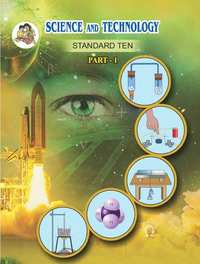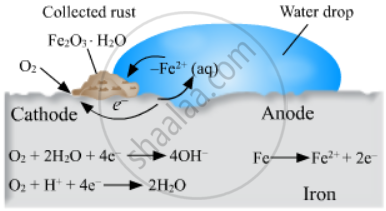# Balbharati solutions for Science and Technology Part 1 10th Standard SSC Maharashtra State Board chapter 3 - Chemical reactions and equations [Latest edition]

#### Chapters## Chapter 3: Chemical reactions and equations

[Pages 45 - 46]

### Balbharati solutions for Science and Technology Part 1 10th Standard SSC Maharashtra State Board Chapter 3 Chemical reactions and equations [Pages 45 - 46]

Q 1.1 | Page 45

Choose the correct option from the bracket and explain the statement giving reason.
(Oxidation, displacement, electrolysis, reduction, zinc, copper, double displacement, decomposition)
To prevent rusting, a layer of ______ metal is applied on iron sheets.

Q 1.2 | Page 45

Choose the correct option from the bracket and explain the statement giving reason.
(Oxidation, displacement, electrolysis, reduction, zinc, copper, double displacement, decomposition)

The conversion of ferrous sulphate to ferric sulphate is ______ reaction.

Q 1.3 | Page 45

Choose the correct option from the given options and explain the statement giving the reason.

When an electric current is passed through acidulated _________ of water takes place.

• Oxidation

• Displacement

• Electrolysis

• Reduction

• zinc

• copper

• Double displacement

• Decomposition

Q 1.4 | Page 45

Choose the correct option from the bracket and explain the statement giving a reason.
(Oxidation, displacement, electrolysis, reduction, zinc, copper, double displacement, decomposition)

Addition of an aqueous solution of ZnSO4 to an aqueous solution of BaCl2 is an example of ....... reaction.

Q 2.1 | Page 45

What is the reaction called when oxidation and reduction take place simultaneously? Explain with one example.

Q 2.2 | Page 45

How can the rate of the chemical reaction, namely, decomposition of hydrogen peroxide be increased?

Q 2.3 | Page 45

Explain the term reactant and product giving examples.

Q 2.4 | Page 45

Explain the types of reaction with reference to oxygen and hydrogen. Illustratre with examples.

Q 2.5 | Page 45

Write the answer to the following.

Explain the similarity and difference in two events, namely adding NaOH to water and adding CaO to water.

Q 3.1 | Page 45

Explain the terms with examples.

Endothermic reaction

Q 3.2 | Page 45

Explain the  terms with examples.

Combination reaction

Q 3.3 | Page 45

Explain the terms with examples.

Balanced equation

Q 3.4 | Page 45

Explain the terms with examples.

Displacement reaction

Q 4.1 | Page 45

Give scientific reason.
When the gas formed on heating limstone is passed through freshly prepared lime water, the lime water turns milky.

Q 4.2 | Page 45

Give scientific reason.

It takes time for pieces of Shahabad tile to disappear in HCl, but its powder disappears rapidly.

Q 4.3 | Page 45

Give scientific reason.

While preparing dilute sulphuric acid from concentrated sulphuric acid in the laboratory, the concentrated sulphuric acid is added slowly to water with constant stirring.

Q 4.4 | Page 45

Give scientific reason.

It is reccommended to use air tight container for storing oil for long time.

Q 5 | Page 45

Observe the following picture a write down the chemical reaction with explanation.Q 6.1 | Page 46

Identify from the reaction the reactants that undergo oxidation and reduction.
Fe + S → FeS

Q 6.2 | Page 46

Identify from the reaction the reactants that undergo oxidation and reduction.

2Ag2O → 4 Ag + O2 ↑

Q 6.3 | Page 46

Identify from the reaction the reactants that undergo oxidation and reduction.

2Mg + O2 → 2MgOa

Q 6.4 | Page 46

Identify from the reaction the reactants that undergo oxidation and reduction.

NiO + H2 → Ni + H2O

Q 7.1 | Page 46

Balance the equation stepwise.
H2S2O7(l) + H2O(l) → H2SO4(l)

Q 7.2 | Page 46

Balance the equation stepwise.

SO2(g) + H2S(aq) → S(s) + H2O(l)

Q 7.3 | Page 46

Balance the equation stepwise.

Ag(s) + HCl(aq) → AgCl ↓+ H2 ↑

Q 7.4 | Page 46

Balance the equation stepwise.

NaOH(aq) + H2SO4(aq) → Na2SO4(aq) + H2O(l)

Q 8.1 | Page 46

Identify the endothermic and exothermic reaction.

HCl + NaOH → NaCl + H2O + heat

Q 8.2 | Page 46

Identify the endothermic and exothermic reaction.

$2 {KClO}_3 \left( s \right) \to^∆ 2KCl\left( s \right) + 3 O_2 \uparrow$

Q 8.3 | Page 46

Identify the endothermic and exothermic reaction.

CaO + H2O → Ca(OH)2 + heat

Q 8.4 | Page 46

Identify the endothermic and exothermic reaction.

$Ca {CO}_3 \left( s \right) \to^∆ CaO\left( s \right) + {CO}_2 \uparrow$

Q 9 | Page 46
 Reactants Products Type of chemical reaction BaCl2(aq) + ZnSO4(aq) H2CO3(aq) Displacement 2AgCl(s) FeSO4(aq) + Cu(s) Combination CuSO4(aq) + Fe(s) BaSO4↓ + ZnCl2(aq) Decomposition H2O(l) + CO2(g) 2Ag(s) + Cl2(g) Double displacement

## Chapter 3: Chemical reactions and equations## Balbharati solutions for Science and Technology Part 1 10th Standard SSC Maharashtra State Board chapter 3 - Chemical reactions and equations

Balbharati solutions for Science and Technology Part 1 10th Standard SSC Maharashtra State Board chapter 3 (Chemical reactions and equations) include all questions with solution and detail explanation. This will clear students doubts about any question and improve application skills while preparing for board exams. The detailed, step-by-step solutions will help you understand the concepts better and clear your confusions, if any. Shaalaa.com has the Maharashtra State Board Science and Technology Part 1 10th Standard SSC Maharashtra State Board solutions in a manner that help students grasp basic concepts better and faster.

Further, we at Shaalaa.com provide such solutions so that students can prepare for written exams. Balbharati textbook solutions can be a core help for self-study and acts as a perfect self-help guidance for students.

Concepts covered in Science and Technology Part 1 10th Standard SSC Maharashtra State Board chapter 3 Chemical reactions and equations are Types of Chemical Reactions, Endothermic and Exothermic Processes, Endothermic and Exothermic Reactions, Rate of Chemical Reaction, Factors Affecting the Rate of a Chemical Reaction, Oxidation and Reduction, Concept of Rancidity, Concept of Chemical Reactions, Chemical Equation, Chemical Equation, Chemical Equation, Chemical Equation, Combination Reaction, Decomposition Reactions, Displacement Reaction, Double Displacement Reaction, Corrosion of Iron (Rusting) and Its Prevention.

Using Balbharati 10th Standard [इयत्ता १० वी] solutions Chemical reactions and equations exercise by students are an easy way to prepare for the exams, as they involve solutions arranged chapter-wise also page wise. The questions involved in Balbharati Solutions are important questions that can be asked in the final exam. Maximum students of Maharashtra State Board 10th Standard [इयत्ता १० वी] prefer Balbharati Textbook Solutions to score more in exam.

Get the free view of chapter 3 Chemical reactions and equations 10th Standard [इयत्ता १० वी] extra questions for Science and Technology Part 1 10th Standard SSC Maharashtra State Board and can use Shaalaa.com to keep it handy for your exam preparation